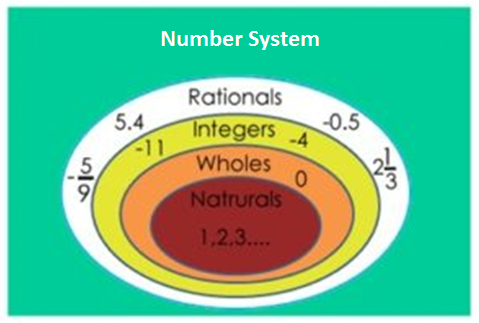# Number System Problems

## Number System Aptitude

Number system is one of the fundamental topics in the quantitative aptitude section of any Exam.

In Decimal number system, there are ten symbols namely 0,1,2,3,4,5,6,7,8 and 9 called digits. A number is denoted by group of these digits called as numerals.

Face value of a digit in a numeral is value of the digit itself. For example in 321, face value of 1 is 1, face value of 2 is 2 and face value of 3 is 3.### Types Of Number

Natural Number: Natural Numbers - n > 0 where n is counting number; [1,2,3...]. Numbers used in counting are termed as natural numbers. Example N={ 1, 2, 3, 4, 5, ....}

Whole Number: Whole Numbers - n ≥ 0 where n is counting number; [0,1,2,3...]. Natural numbers with 0 are termed as whole number. Example W={0, 1, 2, 3, 4, ......}

Prime number: A number which is not divisible by other numbers except the number itself and 1 is termed as prime number. Example 19 is a prime number because it is not divisible by other than 1 and 19

Composite Numbers: Numbers other than one which are not prime are termed as Composite numbers. Example 4, 6, 8, 9, 12

Even Numbers: Numbers which are divisible by 2 ae termed as even numbers. Example 2, 4, 6, 8, 10

Odd Numbers: The number which is not divisible by 2 are termed as odd numbers. Example 3, 7, 9, 11

Consecutive numbers: Numbers increasing by one are termed as consecutive numbers. Example 2, 3, 4, 5, 6, 7, 8, 9, 10, 11, 12, 13

Integers: The set of numbers which consists of Negative numbers and whole numbers is known as Integers. ExampleI= { ....-5, -4, -3, -2, -1, 0, 1, 2, 3, 4..... }

Rational Numbers: The numbers which can be written in the form P / Q , where P and Q are integers and Q not equal to zero is termed as Rational Numbers. Example (1/2), (3/7), (7/11)

Irrational Numbers: The numbers which can not be written in P / Q form are termed as Irrational Numbers 22

Real Numbers: Real number consists of both rational as well as irrational numbers.

Negative Integers: The numbers -1, -2, -3, ... are called negative integers.

Positive Fractions: The numbers(2/3) ,(4/5) ,(7/8) ... are called positive fractions.

Negative Fractions: The numbers -(6/8) ,-(7/19) , -(12/17) ... are called negative fractions.

Note: 0 is the only whole number which is not a natural number. Every natural number is a whole number.

Prime Numbers: Numbers which is divisible by themselves only apart from 1.

Composite Numbers: Non-prime numbers > 1. For example, 4,6,8,9 etc. 1 is neither a prime number nor a composite number. 2 is the only even prime number.

Co-Primes Numbers: Two natural numbers are co-primes if their H.C.F. is 1. For example, (2,3), (4,5) are co-primes.

Rational Numbers: Any number which is a positive or negative integer or fraction, or zero is called a rational number. A rational number is one which can be expressed in the following format (a/b) , where b ≠ 0 and a & b are positive or negative integers. Irrational Numbers: An infinite non-recurring decimal number is known as an irrational number. These numbers cannot be expressed in the form of a proper fraction a/b where b ≠ 0. e.g.√2 , √5 , Π, etc.

Even Numbers: The integers which are divisible by 2 are called even numbers e.g. -4, 0, 2, 16 etc.

Odd Numbers: The integers which are not divisible by 2 are odd numbers e.g. -7, -15, 5, 9 etc.

Prime Numbers: Those numbers, which are divisible only by themselves and 1, are called prime numbers. In other words, a number, which has only two factors, 1 and itself, is called a prime number. e.g. 2, 3, 5, 7, etc.

Note: 2 is the only even prime number.

Composite Number: A number, which has factors other than itself and 1, is called a composite number. e.g. 9, 16, 25...

Note: 1 is neither a composite number nor a prime number.

Real Numbers: The natural numbers, integers, whole numbers, rational numbers and irrational numbers constitute the set of real numbers. Every real number can be represented by a point on a number line.

### Divisible Properties

• Divisibility by 2 - A number is divisible by 2 if its unit digit is 0,2,4,6 or 8.
• Divisibility by 3 - A number is divisible by 3 if sum of its digits is completely divisible by 3.
• Divisibility by 4 - A number is divisible by 4 if number formed using its last two digits is completely divisible by 4.
• Divisibility by 5 - A number is divisible by 5 if its unit digit is 0 or 5.
• Divisibility by 6 - A number is divisible by 6 if the number is divisible by both 2 and 3.
• Divisibility by 8 - A number is divisible by 8 if number formed using its last three digits is completely divisible by 8.
• Divisibility by 9 - A number is divisible by 9 if sum of its digits is completely divisible by 9.
• Divisibility by 10 - A number is divisible by 10 if its unit digit is 0.
• Divisibility by 11 - A number is divisible by 11 if difference between sum of digits at odd places and sum of digits at even places is either 0 or is divisible by 11.

### Tips on Division

• If a number n is divisible by two co-primes numbers a, b then n is divisible by ab.
• (a-b) always divides (an - bn) if n is a natural number.
• (a+b) always divides (an - bn) if n is an even number.
• (a+b) always divides (an + bn) if n is an odd number.